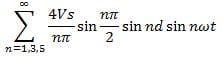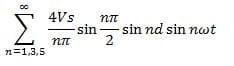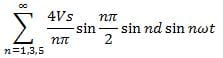Test: Pulse Width Modulation Inverter

# Test: Pulse Width Modulation Inverter

Test Description

## 10 Questions MCQ Test Power Electronics | Test: Pulse Width Modulation Inverter

Test: Pulse Width Modulation Inverter for Electrical Engineering (EE) 2023 is part of Power Electronics preparation. The Test: Pulse Width Modulation Inverter questions and answers have been prepared according to the Electrical Engineering (EE) exam syllabus.The Test: Pulse Width Modulation Inverter MCQs are made for Electrical Engineering (EE) 2023 Exam. Find important definitions, questions, notes, meanings, examples, exercises, MCQs and online tests for Test: Pulse Width Modulation Inverter below.
Solutions of Test: Pulse Width Modulation Inverter questions in English are available as part of our Power Electronics for Electrical Engineering (EE) & Test: Pulse Width Modulation Inverter solutions in Hindi for Power Electronics course. Download more important topics, notes, lectures and mock test series for Electrical Engineering (EE) Exam by signing up for free. Attempt Test: Pulse Width Modulation Inverter | 10 questions in 30 minutes | Mock test for Electrical Engineering (EE) preparation | Free important questions MCQ to study Power Electronics for Electrical Engineering (EE) Exam | Download free PDF with solutions
 1 Crore+ students have signed up on EduRev. Have you?
Test: Pulse Width Modulation Inverter - Question 1

### In the single-pulse width modulation method, the output voltage waveform is symmetrical about __________

Detailed Solution for Test: Pulse Width Modulation Inverter - Question 1

The waveform is a positive in the first half cycle and symmetrical about π/2 in the first half.

Test: Pulse Width Modulation Inverter - Question 2

### The shape of the output voltage waveform in a single PWM is

Detailed Solution for Test: Pulse Width Modulation Inverter - Question 2

Positive and the negative half cycles of the output voltage are symmetrical about π/2 and 3π/2 respectively. The shape of the waveform obtained is called as quasi-square wave.

Test: Pulse Width Modulation Inverter - Question 3

### In the single-pulse width modulation method, the Fourier coefficient an is given by

Detailed Solution for Test: Pulse Width Modulation Inverter - Question 3

As the positive and the negative half cycles are identical the coefficient an = 0.

Test: Pulse Width Modulation Inverter - Question 4

In case of a single-pulse width modulation with the pulse width = 2d, the peak value of the fundamental component of voltage is given by the expression

Detailed Solution for Test: Pulse Width Modulation Inverter - Question 4

For the fundamental component put n = 1.Vo = (4Vs/π) sin (d) sin (ωt)
Hence the peak value is (4Vs/π) sin d.

Test: Pulse Width Modulation Inverter - Question 5

Find the peak value of the fundamental component of voltage with a pulse width of 2d = 90 and Vs = 240 V for single-pulse modulation in a full wave bridge inverter.

Detailed Solution for Test: Pulse Width Modulation Inverter - Question 5

The peak value of the fundamental component of voltage is given by (4Vs/π) sin d.

Test: Pulse Width Modulation Inverter - Question 6

In the single-pulse width modulation method, the output voltage waveform is symmetrical about ____________ in the negative half cycle.

Detailed Solution for Test: Pulse Width Modulation Inverter - Question 6

In the negative half the wave is symmetrical about 3π/2.

Test: Pulse Width Modulation Inverter - Question 7

In the single-pulse width modulation method, the Fourier coefficient bn is given by

Detailed Solution for Test: Pulse Width Modulation Inverter - Question 7

The Fourier analysis is as under:
bn = (2/π) ∫ Vs sin⁡ nωt .d(ωt) , Where the integration would run from (π/2 + d) to (π/2 – d)
2d is the width of the pulse.

Test: Pulse Width Modulation Inverter - Question 8

In the single-pulse width modulation method, when the pulse width of 2d is equal to its maximum value of π radians, then the fundamental component of output voltage is given by

Detailed Solution for Test: Pulse Width Modulation Inverter - Question 8

The Fourier representation of the output voltage is given byPut 2d = π & n = 1.

Test: Pulse Width Modulation Inverter - Question 9

In case of a single-pulse width modulation with the pulse width = 2d, to eliminate the nth harmonic from the output voltage

Detailed Solution for Test: Pulse Width Modulation Inverter - Question 9

To eliminate, the nth harmonic, nd is made equal to π radians, or d = π/n.
From the below expression,when nd = π. sin nd = 0 hence, that output voltage harmonic is eliminated.

Test: Pulse Width Modulation Inverter - Question 10

In case of a single-pulse width modulation with the pulse width = 2d, to eliminate the 3rd harmonic from the output voltage waveform, the value of the pulse width (2d) must be

Detailed Solution for Test: Pulse Width Modulation Inverter - Question 10

To eliminate the nth harmonic, nd = π.
Therefore, d = π/n = π/3 = 60°
Hence, 2d = 120°.

## Power Electronics

5 videos|39 docs|63 tests
 Use Code STAYHOME200 and get INR 200 additional OFF Use Coupon Code
Information about Test: Pulse Width Modulation Inverter Page
In this test you can find the Exam questions for Test: Pulse Width Modulation Inverter solved & explained in the simplest way possible. Besides giving Questions and answers for Test: Pulse Width Modulation Inverter, EduRev gives you an ample number of Online tests for practice

## Power Electronics

5 videos|39 docs|63 tests